# Progress checking Zarankiewicz’s conjecture on the brick factory problem

A previous post presented the fascinating history of the brick factory problem, which wonders about the smallest possible number of rail crossings when connecting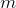kilns and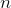storage yards1, which is mathematically modeled by the crossing number of the complete bipartite graph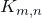.

Proposed by Paul Turán after the Second World War, the first advances on this problem arose in 1952, due to the probabilist Kazimierz Urbanik and the topologist Kazimierz Zarankiewicz. The latter claimed to have solved the problem, stating that the smallest possible number of crossings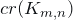was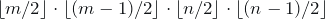and proposing a simple way of attaining this number.

It would be enough to (1) draw thevertices on the x-axis, split as evenly as possible among the strictly positive and the strictly negative semi-axes, (2) proceed analogously with thevertices on the y-axis, and (3) use straight-line segments to join pairs of vertices lying on different axes.

Unfortunately, the proof was found to be incorrect around 1965 2 and, more than sixty years later, Zarankiewicz’s conjecture remains one of the most tantalizing open problems in graph theory.

During these years, some advances have been achieved checking the conjecture for a few values. Zarankiewicz’s proof3, published in 1952, did work for the case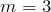. A paper by Kleitman4, in 1970, extended the validity to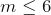and Woodall5, in 1993, checked the cases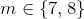and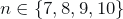with the help of computers.

The aim of this post is to introduce a different kind of progress on checking Zarankiewicz’s conjecture, presented by Christian et al. 6 in a recent paper. For each fixed, the authors manage to determine an integer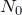(depending on) such that:

If Zarankiewicz’s conjecture holds for the fixedtogether with all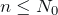,

then it holds for thattogether with every.

In other words, what this paper shows is that, for a given, checking Zarankiewicz’s conjecture reduces to checking a finite number of values for(which is in turn a finite problem). But do not start shouting about it. It turns out that the method is not practical even for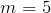.

The expression ofobtained by the authors is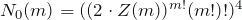,

where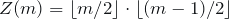(note that this is the part usingin the expression for Zarankiewicz’s conjecture).

This means that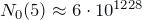, a really big number. The fastest computers today perform in the order of petaFLOPS (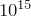operations per second), so even if they could check each case in a single operation, it would take in the order of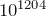years to check if the conjecture holds for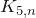with all.

Nevertheless, the result is a remarkable progress in such a tantalizing problem. Although giving full details about the proofs is certainly out of the scope of this post, we can outline the arguments used by Christian et al. In order to do so, we just need to introduce two notions about drawings of.

Consider the vertices in the two classes of the bipartite graph (white and black points in the previous figure) labeled as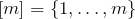and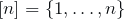. Before introducing the first notion observe that, in any drawing, each vertex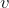of the second class induces a natural cyclic order of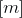, just considering the clockwise cyclic order of the edges incident to it. This cyclic order is the rotation of the vertex in the drawing. The following figure shows a rotation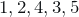.

Note that there are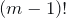possible rotations for a vertex of degree, since this is the number of cyclic permutations of.

The second notion is what Christian et al. call templates. A drawing of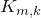is defined to be a template of rankand order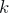if there are no two vertices of degreehaving the same rotation. The authors show that, for each positive integer, there is an optimal drawing ofthat can be obtained (1) from some template of rankand order(2) by duplicating vertices of degreein the template, and (3) so that the crossing number of the resulting optimal drawing ofcan be determined by information contained in the template and the distribution of the duplicate vertices.

A key fact is that, up to isomorphism, the number of templates to consider turns out to be finite.

Then, the authors associate a quadratic program to each template, in such a way that the minimum of the quadratic program provides information about whether, for this template, a drawing ofobtained as above is a counterexample to Zarankiewicz’s conjecture.

In summary, although not being practical today, the paper by Christian et al. opens a way towards checking Zarankiewicz’s conjecture for a given value of. Furthermore, it remarkably finds its place in the list of very few results around this conjecture in the last sixty years.

## References

1. Lowell Beineke and Robin Wilson. The Early History of the Brick Factory Problem. The Mathematical Intelligencer. Volume 32, Issue 2 (June 2010), pages 41-48. http://dx.doi.org/10.1007/s00283-009-9120-4.
2. Richard K. Guy. The decline and fall of Zarankiewicz’s theorem. In: Proof Techniques in Graph Theory (ed. F. Harary), New York: Academic Press (1969), pages 63–69.
3. K. Zarankiewicz. On a problem of P. Turan concerning graphs. Fundamenta Mathematicae. Volume 41 (1954), pages 137–145.
4. Daniel J. Kleitman. The crossing number of. Journal of Combinatorial Theory. Volume 9 (1970), pages 315–323. http://dx.doi.org/10.1016/S0021-9800(70)80087-4
5. Douglas R. Woodall, Cyclic-order graphs and Zarankiewicz’s crossing-number conjecture, Journal of Graph Theory. Volume 17, Issue 6 (December 1993), pages 657–671. http://dx.doi.org/10.1002/jgt.3190170602
6. Christian R., Richter R.B. & Salazar G. (2013). Zarankiewiczʼs Conjecture is finite for each fixed m, Journal of Combinatorial Theory, Series B, 103 (2) 237-247. DOI: 10.1016/j.jctb.2012.11.001

## 1 comment

• […] escribí para Mapping Ignorance y que llegó hasta reddit. En el mismo blog, en la entrada Progress checking Zarankiewicz’s conjecture on the brick factory problem, puedes encontrar algunos progresos recientes relacionados con este problema. Aunque no han […]